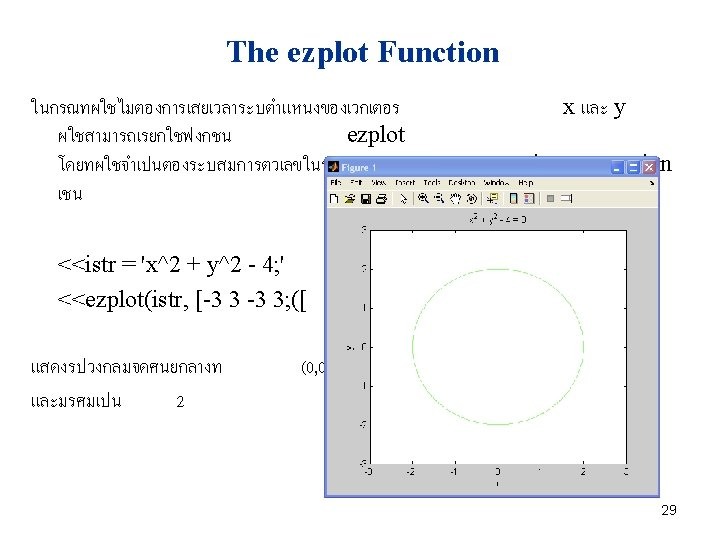# Changing the Default Format MATLAB 4 format Format

• Slides: 31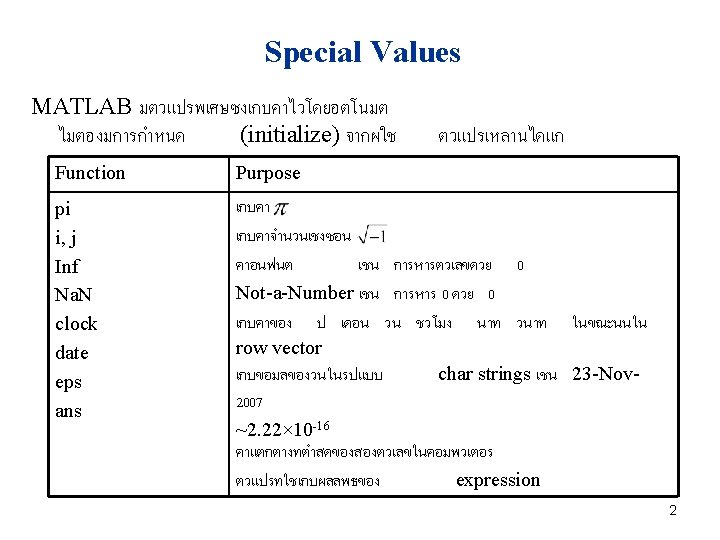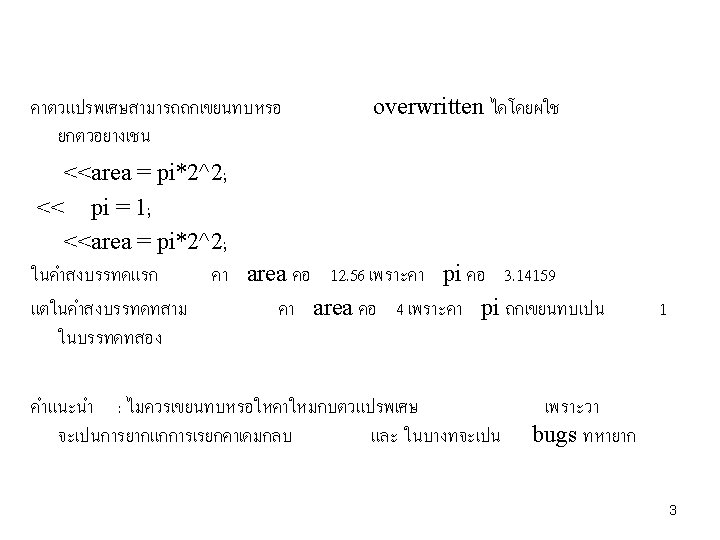Changing the Default Format MATLAB โดยปกตจะแสดงคาของตวเลขกบจดทศนยม 4 ตำแหนง หากผใชตองการเปลยนการแสดงคา กสามารถทำไดดวยการใชคำสง format ซงมคำสงดงน Format Command format short format long format short e format short g format long e format long g Results 4 digits after decimal (default format) 14 digits after decimal 5 digits plus exponent 5 total digits with or without exponential 15 digits plus exponent 15 total digits with or without exponent Example 12. 3457 12. 34567890123456 1. 2346 e+001 12. 346 1. 23456789012345 e+001 12. 3456789012346 4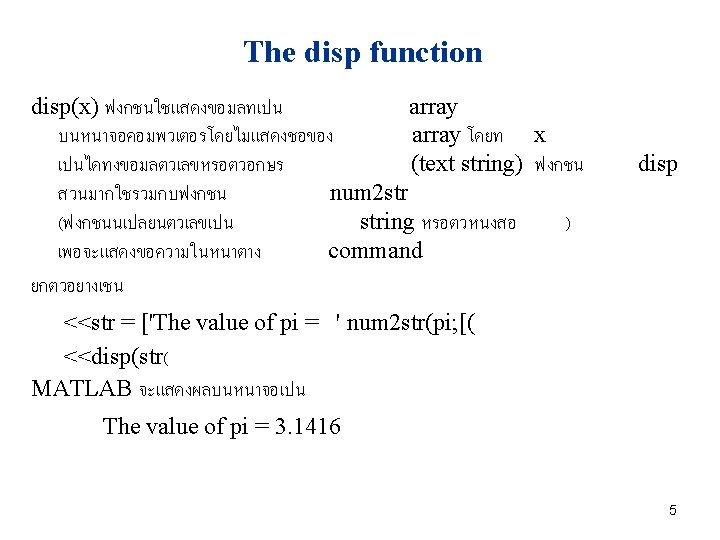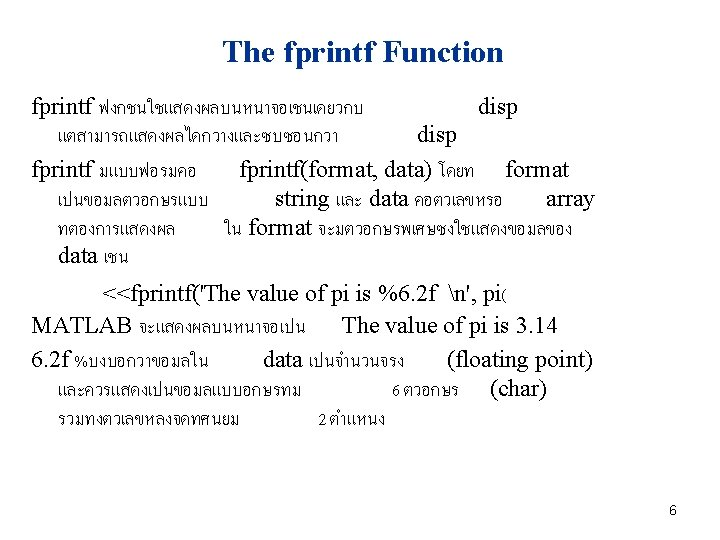Special Characters in fprintf Format Strings ตวอยางของตวอกษรพเศษทใชใน Format String %d %e %f n t format ของคำสง fprintf มดงน Results Display value as an integer Display value in exponential format Display value in floating point format Skip to a new line Horizontal tab 7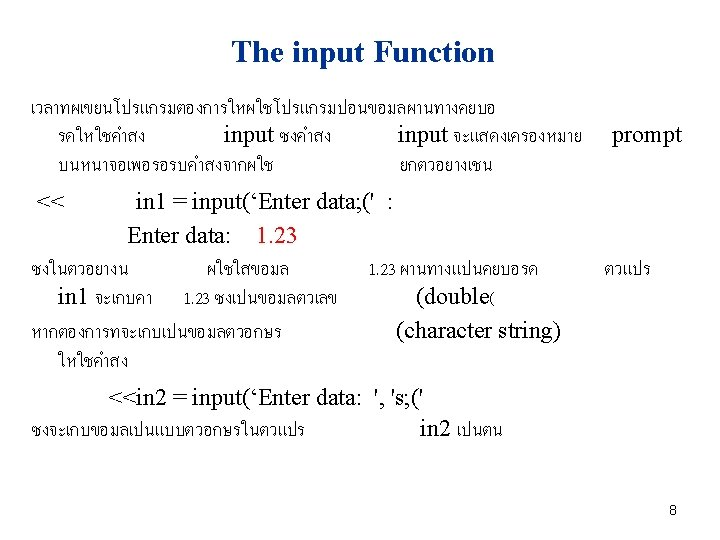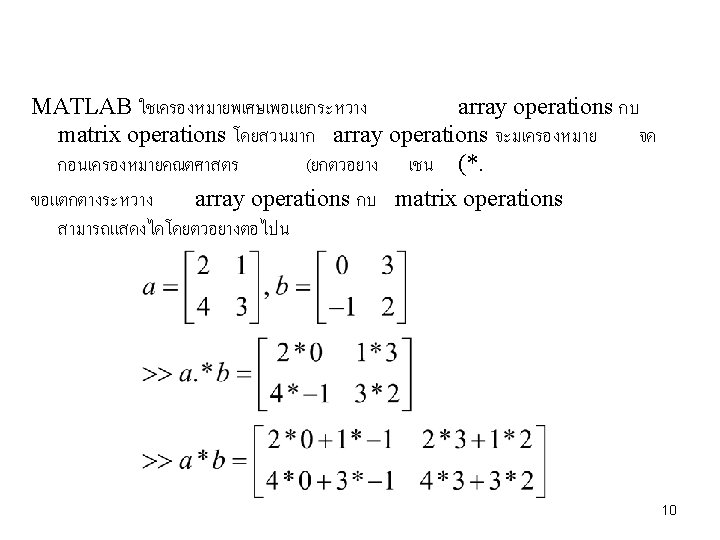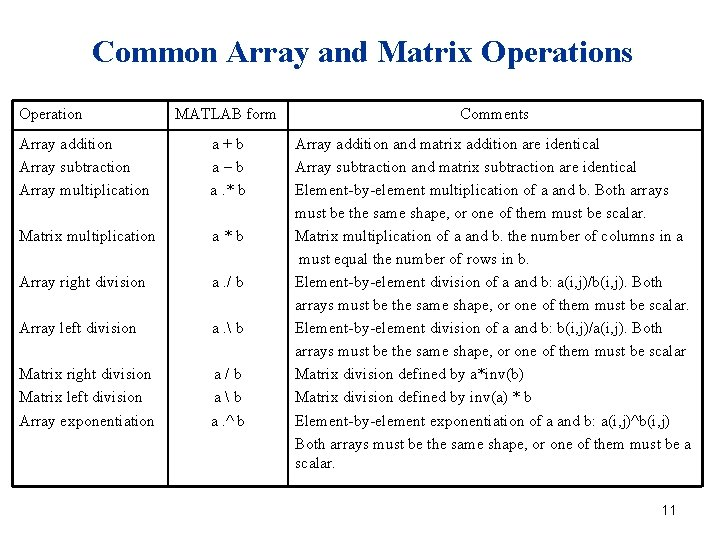Common Array and Matrix Operations Operation MATLAB form Comments Array addition Array subtraction Array multiplication a+b a–b a. * b Matrix multiplication a*b Array right division a. / b Array left division a. b Matrix right division Matrix left division Array exponentiation a/b ab a. ^ b Array addition and matrix addition are identical Array subtraction and matrix subtraction are identical Element-by-element multiplication of a and b. Both arrays must be the same shape, or one of them must be scalar. Matrix multiplication of a and b. the number of columns in a must equal the number of rows in b. Element-by-element division of a and b: a(i, j)/b(i, j). Both arrays must be the same shape, or one of them must be scalar. Element-by-element division of a and b: b(i, j)/a(i, j). Both arrays must be the same shape, or one of them must be scalar Matrix division defined by a*inv(b) Matrix division defined by inv(a) * b Element-by-element exponentiation of a and b: a(i, j)^b(i, j) Both arrays must be the same shape, or one of them must be a scalar. 11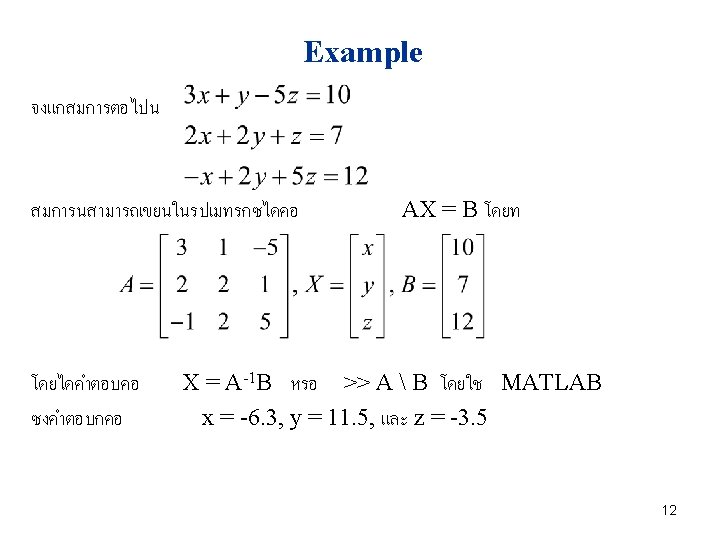Mathematical Functions Function abs(x) acos(x) angle(x) asin(x) atan(x) cos(x) exp(x) log(x) [value, index] = max(x) [value, index] = min(x) mod(x, y) sin(x) sqrt(x) tan(x) Description Calculates |x| Calculates cos-1 x Returns the phase angle of the complex value x, in radians Calculates sin-1 x Calculates tan-1 x Calculates cos x, with x in radians Calculates ex Calculates the natural logarithm logex Returns the max value in vector x, and the location of that value Returns the min value in vector x, and the location of that value Returns the remainder of x/y Calculates sin x, with x in radians Calculates the square root of x Calculates tan x, with x in radians 13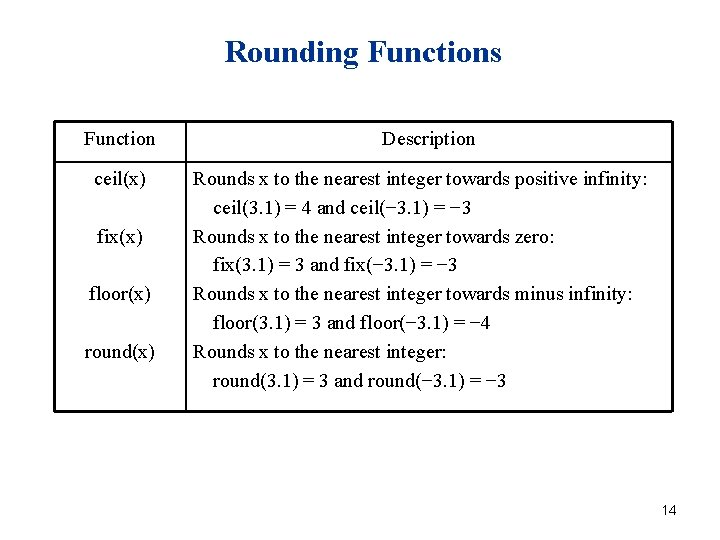Rounding Functions Function ceil(x) fix(x) floor(x) round(x) Description Rounds x to the nearest integer towards positive infinity: ceil(3. 1) = 4 and ceil(− 3. 1) = − 3 Rounds x to the nearest integer towards zero: fix(3. 1) = 3 and fix(− 3. 1) = − 3 Rounds x to the nearest integer towards minus infinity: floor(3. 1) = 3 and floor(− 3. 1) = − 4 Rounds x to the nearest integer: round(3. 1) = 3 and round(− 3. 1) = − 3 14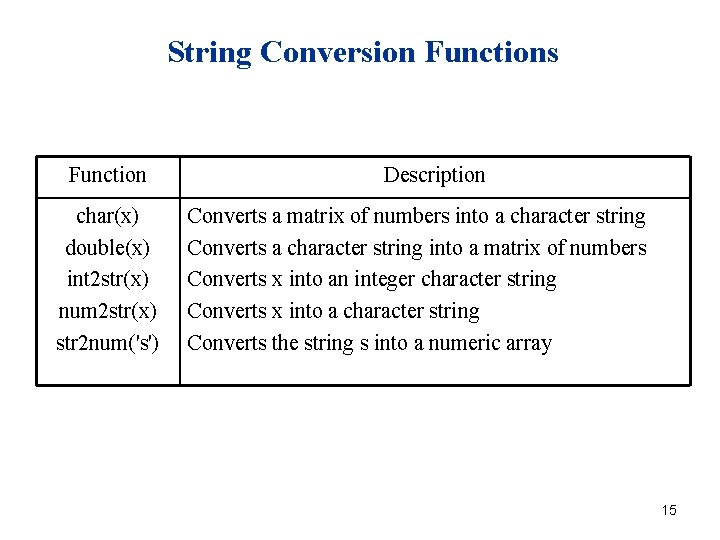String Conversion Functions Function char(x) double(x) int 2 str(x) num 2 str(x) str 2 num('s') Description Converts a matrix of numbers into a character string Converts a character string into a matrix of numbers Converts x into an integer character string Converts x into a character string Converts the string s into a numeric array 15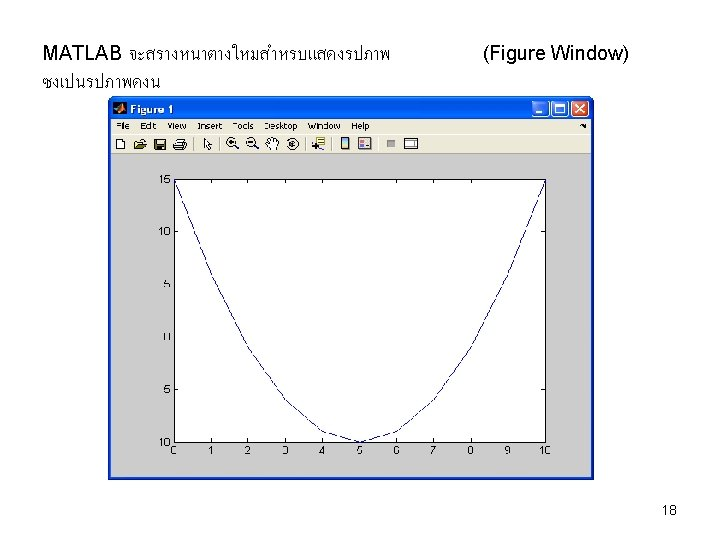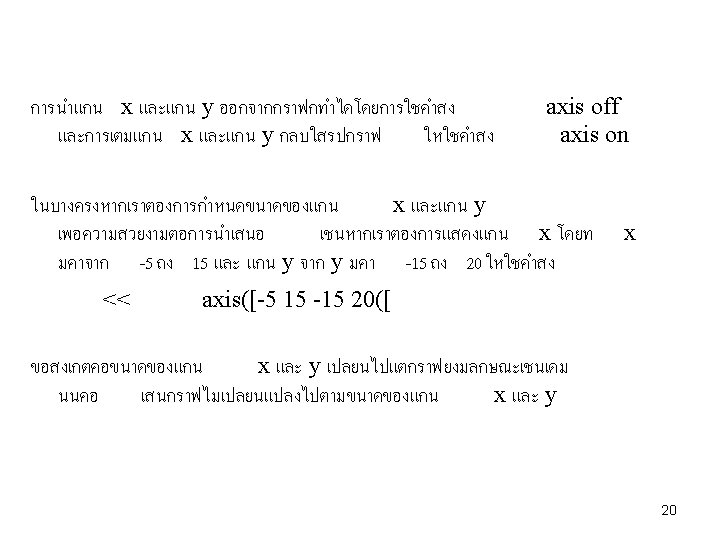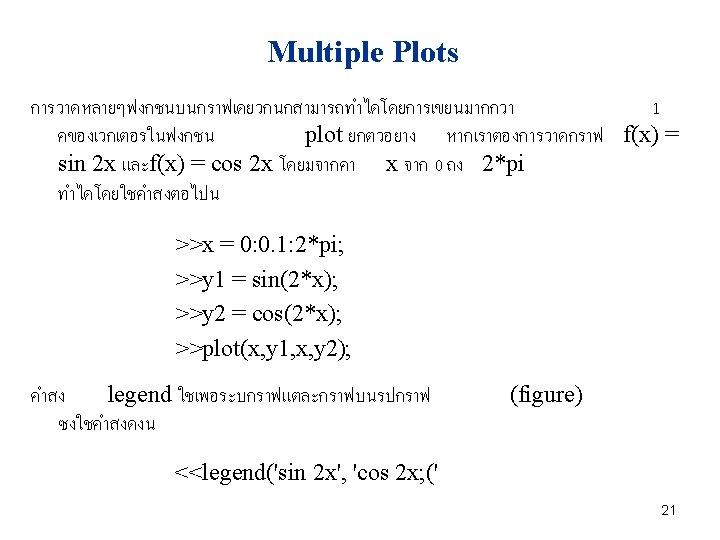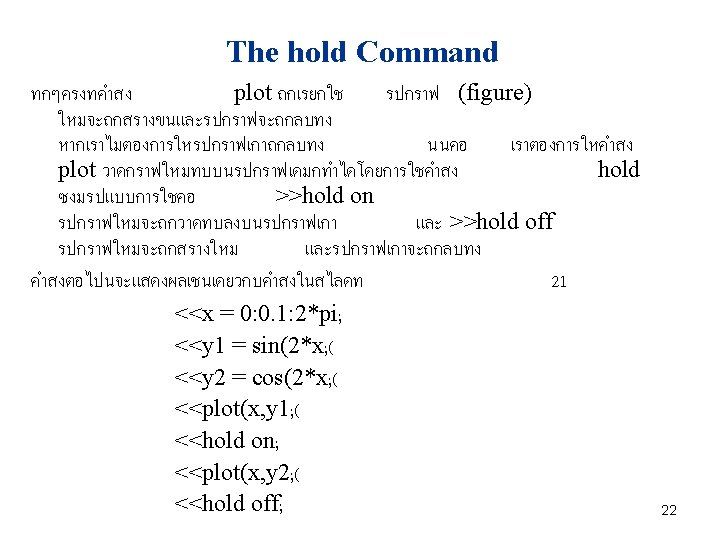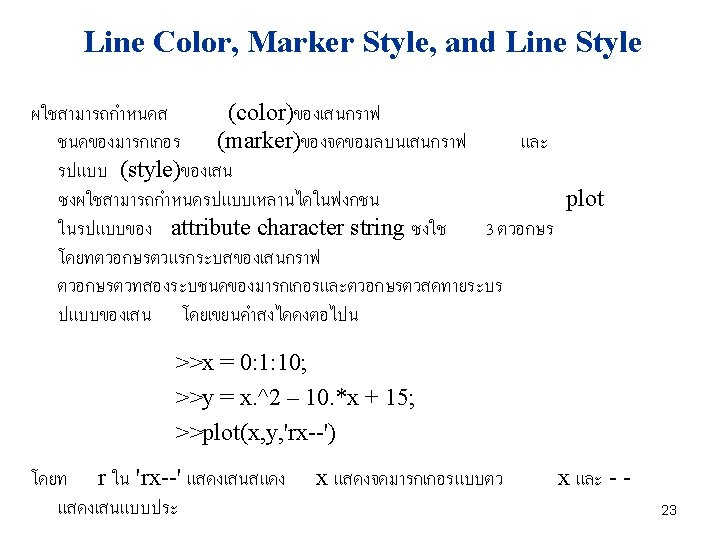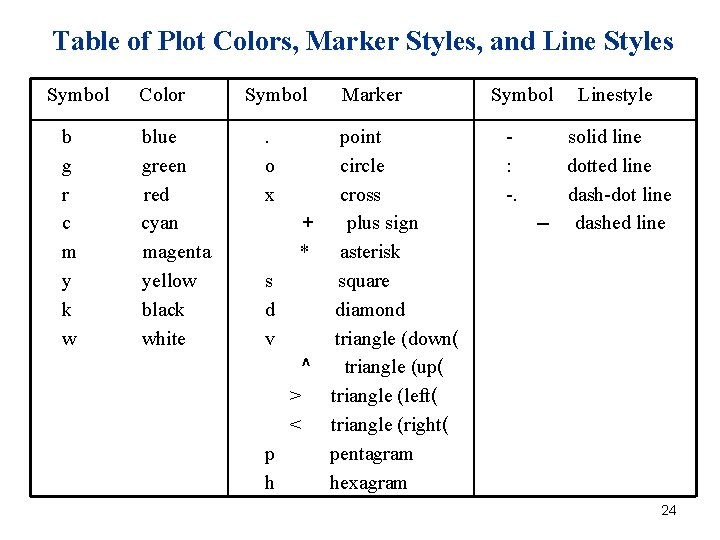Table of Plot Colors, Marker Styles, and Line Styles Symbol b g r c m y k w Color blue green red cyan magenta yellow black white Symbol. o x Marker point circle cross + plus sign * asterisk s square d diamond v triangle (down( ^ triangle (up( > triangle (left( < triangle (right( p pentagram h hexagram Symbol : -. Linestyle solid line dotted line dash-dot line -- dashed line 24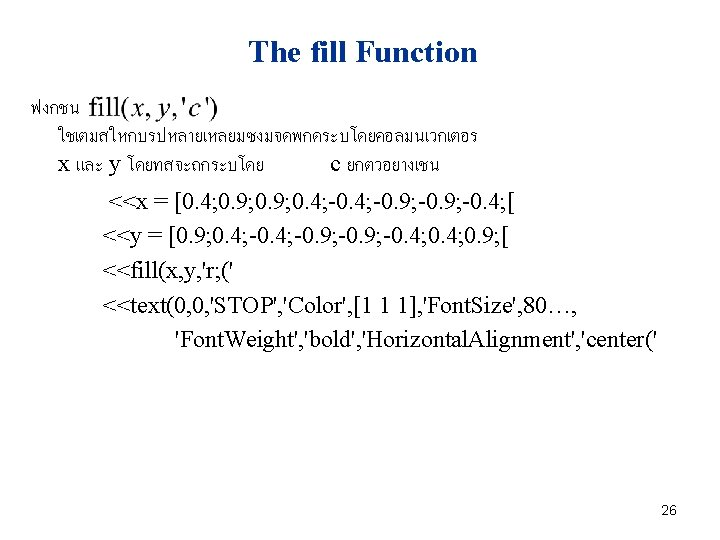27